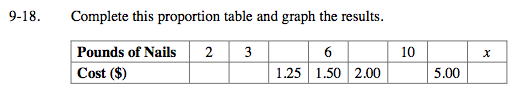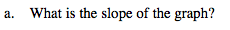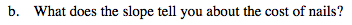### Home > MC2 > Chapter 9 > Lesson 9.1.2 > Problem9-18

9-18.If 6 pounds of nails costs \$1.50, how much does 3 pounds of nails cost?

$\frac{6}{1.50}\left(\frac{?}{?}\right)=\frac{3}{x}$

Solve for x.

x = 0.75
Now set up proportions for all other values in the table.$\frac{0.75 - 0.50}{3 - 2} = ?$The slope tells you how much nails cost per pound.

Complete the table in the eTool below to graph the results.
Click the link at right for the full version of the eTool: MC2 -18 HW eTool